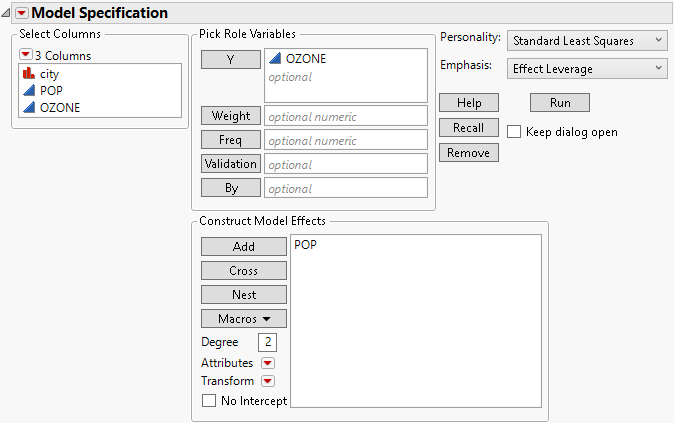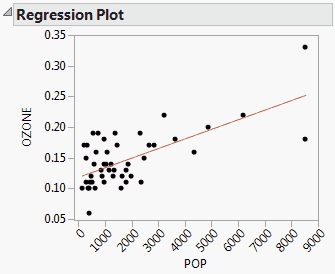Publication date: 04/12/2021

## Simple Linear Regression

Effects to be entered: X

1. In the Select Columns list, select X.

2. Click Add.

#### Example of Simple Linear Regression Model

You are interested in the relationship between the population in thousands of the given city, and ozone levels. Ozone is the response of interest, and POP is the continuous model effect.

1. Select Help > Sample Data Library and open Polycity.jmp.

2. Select Analyze > Fit Model.

3. In the Select Columns list, select Ozone and click Y.

4. In the Select Columns list, select POP.

5. Click Add.

Figure 2.4 Fit Model Window for Simple Linear RegressionWhen you click Run, the Fit Least Squares report appears, showing various results, including a Regression Plot. The Regression Plot shows the data and a simple linear regression model fit to the data.

Figure 2.5 Model Fit for Simple Linear RegressionWant more information? Have questions? Get answers in the JMP User Community (community.jmp.com).